true
true

Ashwin Mohan

Kodambakkam, Chennai, India - 600033# Ashwin Mohan

Kodambakkam, Chennai, India - 600033.

3.6

UrbanPro Rating

Details verified of Ashwin Mohan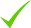IdentityEducation

Know how UrbanPro verifies Tutor details

Identity is verified based on matching the details uploaded by the Tutor with government databases.

## Overview

I have a thorough grounding on the fundamentals in Mathematics and Physics from high school and from courses pursued at university, with excellent grades in both. My pursuit of education in the sciences has always been motivated by a desire to teach, a desire that has been present in me for many years. I have been teaching basic science to small classrooms at Ananya, a learning center for dyslexic students that is under the ambit of Madras Dyslexia Association. At university level, I was a Teaching Assistant for a course on Electromagnetic Theory where I was responsible for holding doubt clearance sessions, in addition to my responsibilities of designing and correcting worksheets and assignments.
Using my experience as a TA, I started a new venture in association with the physics department at my university called the H-bar evening lecture series. I conceptualised the course as an introduction to quantum mechanics for students from all disciplines who wanted to know more about the subject and may not have the chance to pursue a formal course in college. The course syllabus was designed and taught by me over a period of 5 months for nearly 100 registered students.
My teaching experience has spanned a wide range of audiences and class sizes across a diverse spectrum of syllabi. With a keen sense of academic requirements, the classes can be tailored to suit the student's needs.

English

Tamil

## Education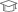Birla Institute of Technology and Science

Master of Science (M.Sc.)

Kodambakkam, Chennai, India - 600033

## Verified Info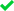Phone Verified

Report this Profile

Is this listing inaccurate or duplicate? Any other problem?

Type the letters as shown below *

Please enter the letters as show below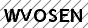Summer Camp classes

Class LocationStudent's HomeTutor's HomeOnline (video chat via skype, google hangout etc)

Years of Experience in Summer Camp classes

1

Type of camp conducted

Math & Science

Age groups catered to

Above 10, Above 13

## Reviews

this is test message this is test message this is test message this is test message this is test message this is test message this is test message

No Reviews yet! Be the first one to Review

## FAQs

1. Which classes do you teach?

I teach BSc Tuition, Class 10 Tuition, Class 11 Tuition, Class 12 Tuition, Class 9 Tuition, MSc Tuition and Summer Camp Classes.

2. Do you provide a demo class?

No, I don't provide a demo class.

3. How many years of experience do you have?

I have been teaching for 1 year.

## Answers by Ashwin Mohan (2)

Let's break this down so that you have a full solution instead of just an answer. We will make use of the two elementary trigonometric identities. d(sin(x))/dx = cos(x) d(cos(x))/dx = -sin(x) From the chain rule we know that d(f(kx))/dx = kdf(kx)/dx for a constant multiple k. To take a simple example,... ...more
Let's break this down so that you have a full solution instead of just an answer. We will make use of the two elementary trigonometric identities. d(sin(x))/dx = cos(x) d(cos(x))/dx = -sin(x) From the chain rule we know that d(f(kx))/dx = kdf(kx)/dx for a constant multiple k. To take a simple example, if we had f(x) = x^{2}; and took k= 2 d(2x^{2})/dx = 2d(x^{2})/dx = 2 x 2x = 4x. So we have d(sin3x)/dx = 3d(sin3x)/dx = 3 cos(3x) d(cos4x)/dx = 4d(cos(4x))/dx = -4sin(4x) The overall answer then, as many of other replies rightly conclude, is 3cos3x-4sin4x.
Dislike Bookmark

In some sense, the answer to this is usually no. For starters, there are two things that you can note from the standard definition of prime numbers, which I write down below in slightly simpler form. A natural number is a prime number if it's only factor are the unit (1) and the number itself. Following... ...more
In some sense, the answer to this is usually no. For starters, there are two things that you can note from the standard definition of prime numbers, which I write down below in slightly simpler form. A natural number is a prime number if it's only factor are the unit (1) and the number itself. Following on from that, zero fails on two counts. 1) It is not a part of the set of natural numbers. 2) Ignoring the argument that 0 doesn't belong to the natural numbers, 0 = n x 0 for all numbers n (1 x 0 = 2 x 0 = ... = 0). Therefore, one could argue that all natural numbers are 'factors' for 0. So, it can be argued that 0 isn't prime. It's also not very useful to consider 0 as a prime number, which involves a little analysis into basic number theory but I won't go into that here, do message me if you require additional comments on that.
Dislike Bookmark
Summer Camp classes 3.6

Class LocationStudent's HomeTutor's HomeOnline (video chat via skype, google hangout etc)

Years of Experience in Summer Camp classes

1

Type of camp conducted

Math & Science

Age groups catered to

Above 10, Above 13

Class 9 Tuition 3.6

Class LocationStudent's HomeTutor's HomeOnline (video chat via skype, google hangout etc)

Years of Experience in Class 9 Tuition

1

Board

CBSE, State

CBSE Subjects taught

Science, Mathematics, English

Taught in School or College

Yes

State Syllabus Subjects taught

English, Mathematics, Science

Class 10 Tuition 3.6

Class LocationStudent's HomeTutor's HomeOnline (video chat via skype, google hangout etc)

Years of Experience in Class 10 Tuition

1

Board

CBSE, State

CBSE Subjects taught

Science, Mathematics, English

Taught in School or College

Yes

State Syllabus Subjects taught

English, Mathematics, Science

Class 11 Tuition 3.6

Class LocationStudent's HomeTutor's HomeOnline (video chat via skype, google hangout etc)

Years of Experience in Class 11 Tuition

1

Board

CBSE

CBSE Subjects taught

Physics, English, Mathematics

Taught in School or College

Yes

Class 12 Tuition 3.6

Class LocationStudent's HomeTutor's HomeOnline (video chat via skype, google hangout etc)

Years of Experience in Class 12 Tuition

1

Board

CBSE

CBSE Subjects taught

Physics, English, Mathematics

Taught in School or College

Yes

MSc Tuition 3.6

Class LocationStudent's HomeTutor's HomeOnline (video chat via skype, google hangout etc)

Years of Experience in MSc Tuition

1

Subject

Physics, Mathematics

Taught in School or College

Yes

BSc Tuition 3.6

Class LocationStudent's HomeTutor's HomeOnline (video chat via skype, google hangout etc)

Years of Experience in BSc Tuition

1

BSc Physics Subjects

Electromagnetic Theory, Quantum Mechanics, Electricity and Magnetism, Oscillations and Waves, Mathematics, Mathematical Physics, Numerical Analysis

Type of class

Regular Classes

Class strength catered to

Group Classes, One on one/ Private Tutions

Taught in School or College

Yes

BSc Branch

BSc Physics, BSc Mathematics

BSc Mathematics Subjects

Algebra, Calculus, Physics

this is test message this is test message this is test message this is test message this is test message this is test message this is test message

No Reviews yet! Be the first one to Review

## Answers by Ashwin Mohan (2)

Let's break this down so that you have a full solution instead of just an answer. We will make use of the two elementary trigonometric identities. d(sin(x))/dx = cos(x) d(cos(x))/dx = -sin(x) From the chain rule we know that d(f(kx))/dx = kdf(kx)/dx for a constant multiple k. To take a simple example,... ...more
Let's break this down so that you have a full solution instead of just an answer. We will make use of the two elementary trigonometric identities. d(sin(x))/dx = cos(x) d(cos(x))/dx = -sin(x) From the chain rule we know that d(f(kx))/dx = kdf(kx)/dx for a constant multiple k. To take a simple example, if we had f(x) = x^{2}; and took k= 2 d(2x^{2})/dx = 2d(x^{2})/dx = 2 x 2x = 4x. So we have d(sin3x)/dx = 3d(sin3x)/dx = 3 cos(3x) d(cos4x)/dx = 4d(cos(4x))/dx = -4sin(4x) The overall answer then, as many of other replies rightly conclude, is 3cos3x-4sin4x.
Dislike Bookmark

In some sense, the answer to this is usually no. For starters, there are two things that you can note from the standard definition of prime numbers, which I write down below in slightly simpler form. A natural number is a prime number if it's only factor are the unit (1) and the number itself. Following... ...more
In some sense, the answer to this is usually no. For starters, there are two things that you can note from the standard definition of prime numbers, which I write down below in slightly simpler form. A natural number is a prime number if it's only factor are the unit (1) and the number itself. Following on from that, zero fails on two counts. 1) It is not a part of the set of natural numbers. 2) Ignoring the argument that 0 doesn't belong to the natural numbers, 0 = n x 0 for all numbers n (1 x 0 = 2 x 0 = ... = 0). Therefore, one could argue that all natural numbers are 'factors' for 0. So, it can be argued that 0 isn't prime. It's also not very useful to consider 0 as a prime number, which involves a little analysis into basic number theory but I won't go into that here, do message me if you require additional comments on that.
Dislike Bookmark

Ashwin Mohan conducts classes in BSc Tuition, Class 10 Tuition and Class 11 Tuition. Ashwin is located in Kodambakkam, Chennai. Ashwin takes at students Home, Regular Classes- at his Home and Online Classes- via online medium. He has 1 years of teaching experience . Ashwin has completed Master of Science (M.Sc.) from Birla Institute of Technology and Science. He is well versed in English and Tamil.

•• Want to learn from Ashwin Mohan?

• Contact Now
X

Recommended Profiles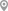Guindy, ChennaiMehdipatnam, HyderabadKodambakkam, ChennaiKasba Peth, PuneMunirka, DelhiKothanur, Bangalore

X

Let us shortlist and give the best tutors and institutes.

or

Send Enquiry to Ashwin Mohan

Let Ashwin Mohan know you are interested in their class